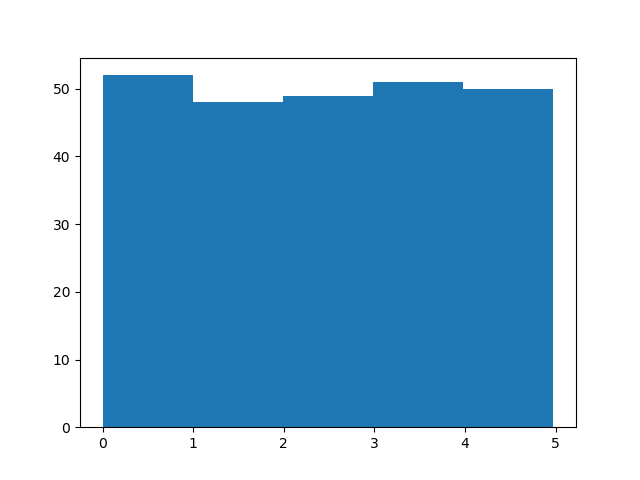## Python Tutorial

Python HOME Python Intro Python Get Started Python Syntax Python Comments Python Variables Python Data Types Python Numbers Python Casting Python Strings Python Booleans Python Operators Python Lists Python Tuples Python Sets Python Dictionaries Python If...Else Python While Loops Python For Loops Python Functions Python Lambda Python Arrays Python Classes/Objects Python Inheritance Python Iterators Python Polymorphism Python Scope Python Modules Python Dates Python Math Python JSON Python RegEx Python PIP Python Try...Except Python User Input Python String Formatting

## File Handling

Python File Handling Python Read Files Python Write/Create Files Python Delete Files

## Python Modules

NumPy Tutorial Pandas Tutorial SciPy Tutorial Django Tutorial

## Python Matplotlib

Matplotlib Intro Matplotlib Get Started Matplotlib Pyplot Matplotlib Plotting Matplotlib Markers Matplotlib Line Matplotlib Labels Matplotlib Grid Matplotlib Subplot Matplotlib Scatter Matplotlib Bars Matplotlib Histograms Matplotlib Pie Charts

## Machine Learning

Getting Started Mean Median Mode Standard Deviation Percentile Data Distribution Normal Data Distribution Scatter Plot Linear Regression Polynomial Regression Multiple Regression Scale Train/Test Decision Tree Confusion Matrix Hierarchical Clustering Logistic Regression Grid Search Categorical Data K-means Bootstrap Aggregation Cross Validation AUC - ROC Curve K-nearest neighbors

## Python MySQL

MySQL Get Started MySQL Create Database MySQL Create Table MySQL Insert MySQL Select MySQL Where MySQL Order By MySQL Delete MySQL Drop Table MySQL Update MySQL Limit MySQL Join

## Python MongoDB

MongoDB Get Started MongoDB Create Database MongoDB Create Collection MongoDB Insert MongoDB Find MongoDB Query MongoDB Sort MongoDB Delete MongoDB Drop Collection MongoDB Update MongoDB Limit

## Python Reference

Python Overview Python Built-in Functions Python String Methods Python List Methods Python Dictionary Methods Python Tuple Methods Python Set Methods Python File Methods Python Keywords Python Exceptions Python Glossary

## Module Reference

Random Module Requests Module Statistics Module Math Module cMath Module

## Python How To

Remove List Duplicates Reverse a String Add Two Numbers

## Python Examples

Python Examples Python Compiler Python Exercises Python Quiz Python Bootcamp Python Certificate

# Machine Learning - Data Distribution

## Data Distribution

Earlier in this tutorial we have worked with very small amounts of data in our examples, just to understand the different concepts.

In the real world, the data sets are much bigger, but it can be difficult to gather real world data, at least at an early stage of a project.

### How Can we Get Big Data Sets?

To create big data sets for testing, we use the Python module NumPy, which comes with a number of methods to create random data sets, of any size.

### Example

Create an array containing 250 random floats between 0 and 5:

import numpy

x = numpy.random.uniform(0.0, 5.0, 250)

print(x)
Try it Yourself »

## Histogram

To visualize the data set we can draw a histogram with the data we collected.

We will use the Python module Matplotlib to draw a histogram.

Learn about the Matplotlib module in our Matplotlib Tutorial.

### Example

Draw a histogram:

import numpy
import matplotlib.pyplot as plt

x = numpy.random.uniform(0.0, 5.0, 250)

plt.hist(x, 5)
plt.show()

### Result:Run example »

### Histogram Explained

We use the array from the example above to draw a histogram with 5 bars.

The first bar represents how many values in the array are between 0 and 1.

The second bar represents how many values are between 1 and 2.

Etc.

Which gives us this result:

• 52 values are between 0 and 1
• 48 values are between 1 and 2
• 49 values are between 2 and 3
• 51 values are between 3 and 4
• 50 values are between 4 and 5

Note: The array values are random numbers and will not show the exact same result on your computer.

## Big Data Distributions

An array containing 250 values is not considered very big, but now you know how to create a random set of values, and by changing the parameters, you can create the data set as big as you want.

### Example

Create an array with 100000 random numbers, and display them using a histogram with 100 bars:

import numpy
import matplotlib.pyplot as plt

x = numpy.random.uniform(0.0, 5.0, 100000)

plt.hist(x, 100)
plt.show()
Run example »

W3Schools is optimized for learning and training. Examples might be simplified to improve reading and learning. Tutorials, references, and examples are constantly reviewed to avoid errors, but we cannot warrant full correctness of all content. While using W3Schools, you agree to have read and accepted our terms of use, cookie and privacy policy.